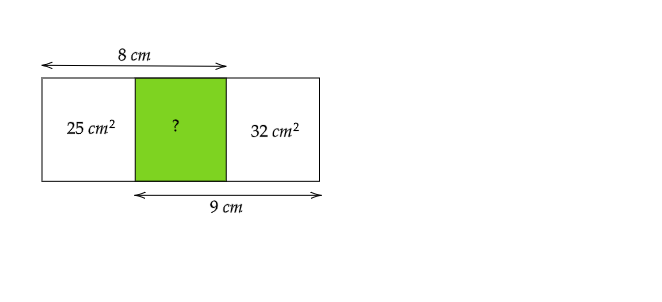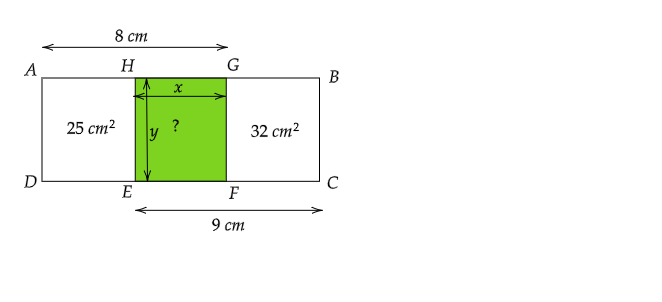"
">

# Find the area of the green figure:"

To do: To find the are of the green figure.

Solution:Let $x$ and $y$ are the sides of the green figure.

In rectangle $FCBG$:

Length$=FC=CE-EF=9-x$

Breadth$=FG=HE=y$

Area of the rectangle $FCBG=(9-x)y=32$     [given area$=32\ cm^2$]

$\Rightarrow 9y-xy=32$

$\Rightarrow xy=9y-32\ ...............\ ( i)$

In rectangle $DEHA$

Length of the rectangle $=AH=AG-GH=8-x$

Breadth$=AD=HE=y$

Area of the rectangle $DEHA=( 8-x)y=25$

$\Rightarrow 8y-xy=25$

$\Rightarrow 8y-9y+32=25$                 [$\because xy=9y-32$ from $( i)$]

$\Rightarrow -y=25-32=-7$

$\Rightarrow y=7$, Put this value in (i),

$7x=9\times7-32$

$\Rightarrow 7x=63-32$

$\Rightarrow 7x=31$

$\Rightarrow x=\frac{31}{7}$.

Therefore, area of the green figure$=xy=7\times\frac{31}{7}=31\ cm^2$

Thus, area of the green figure is $31\ cm^2$.

Updated on: 10-Oct-2022

37 Views